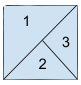New update is available. Click here to update.
Last Updated: 19 Mar, 2021
##### Regions Cut By Slashes
Moderate
Problem statement#### This time our favorite Ninja wants to buy a house. But they are unable to count the number of rooms in the house. So they need your help to count the number of rooms.

##### Note:
``````A house is represented by a matrix where “\” and “/” represent a wall and blank space is empty space. We will consider two rooms different if we will be unable to reach another room.
``````

#### Your task is to return the number of rooms. For a better explanation, see the example.

##### Example:
``````Input:
[
“ /”
“/\”
]

Output: 3

Explanation: 2 X 2 house is shown below:
``````##### Input Format:
``````The first line of input contains an integer 'T' representing the number of test cases.

The first line of each test case contains the ‘N’ number of strings.

The next ‘N’ lines of each test case contain a single string of length ‘N’.
``````
##### Output Format:
``````For each test case, return the number of the separate rooms.

The output of each test case will be printed in a separate line.
``````
##### Note:
``````You do not need to print anything, it has already been taken care of. Just implement the given function.
``````
##### Constraints:
``````1 <= T <= 5
2 <= N <= 30

Time limit: 1 sec
``````Approaches

## 01ApproachFirst, we will convert our given matrix to a more convenient one so that it would be easy for us to find the number of components in the graph(i.e., Number of rooms). Then we can find the number of components either by depth-first search or disjoint union.

Just magnify each box of the house three times.

The steps are as follows:

• Declare a new matrix ‘newhouse’ of size 3 * ‘N’ where ‘N’ is the size of the given matrix.
• Initialize matrix with all zeros.
• Iterate through the given ‘N’ strings and fill the ‘newHouse’ as follows:
• Let current character is’\’ and its position is (i,j), then
• We will assign 1 to (3 * i, 3 * j), (3 * i + 1, 3 * j + 1), (3 * i + 2,3 * j + 2)
• Now, if our current character is ‘/’ and its position is (i , j), then
• We will assign 1 to (3 * i, 3 * j + 2), (3 * i + 1, 3 * j + 1), (3 * i + 2, 3 * j)

After this we will count the number of components by using depth-first search or union-find.

void ‘DFS’(int ‘i’, int ‘j’, vector<vector<int>> &‘newHouse’, int ‘N’)

{

newHouse[i][j] := 1

We will call dfs again on the following values ('i' + 1, ‘j’), ('i' - 1, ‘j’), ('i', ‘j’ + 1), ('i', ‘j’ - 1), if these values exist in the table and ‘newHouse’ value is 0.

dfs(a, b, ’newHouse’, ’N’);

}

We are using DFS because it is easy to understand and implement.